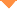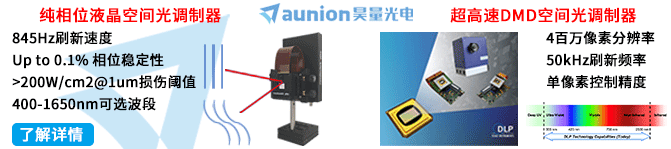首页 > 论文 > 激光与光电子学进展 > 57卷 > 15期(pp：153001--1)

Combination of Fractional Order Differential and Machine Learning Algorithm for Spectral Estimation of Soil Organic Carbon Content

• 摘要• 论文信息• 参考文献• 被引情况• PDF全文Abstract

In this study, 96 surface soil samples are obtained from the typical oasis of the Ugan-Kuqa River in the Xinjiang Uyghur Autonomous Region and their spectral reflectance and soil organic carbon (SOC) content are evaluated. Using fractional order differential technique (with an order value range of 0-2 and a step size of 0.2) is combined with five machine learning algorithms, including the extreme learning machine, random forest, multiple adaptive regression spline function, elastic network regression, and gradient lifting regression tree (GBRT) algorithms, and high-precision estimation of SOC content. The experimental results show that the pretreatment effect obtained using a fractional order differential is better than that obtained using an integer order differential. The correlation at a specific band is significantly improved, and the maximum correlation is enhanced by approximately 0.220. In case of the GBRT, the verification concentration determination coefficient is 0.878 and the relative analysis error is 3.142, indicating that this type of integrated learning is superior to other models of different orders. GBRT based on a 1.6-order spectral reflectance should be used to estimate the SOC content of the oasis in arid areas. Thus, a new scheme based on the combination of visible light-near infrared(VIS-NIR)with the fractional order differential technology and machine learning algorithms is proposed in this study to improve the accuracy of the model used for estimating the SOC content of the oasis in arid areas.【1】Sakschewski B, von Bloh W, Boit A, et al. Resilience of Amazon forests emerges from plant trait diversity [J]. Nature Climate Change. 2016, 6(11): 1032-1036.

【3】Viscarra Rossel R A, Behrens T, Ben-Dor E, et al. A global spectral library to characterize the world''''s soil [J]. Earth-Science Reviews. 2016, 155: 198-230.

【4】Fang X, Guo X L, Zhang C, et al. Contributions of climate change to the terrestrial carbon stock of the arid region of China: a multi-dataset analysis [J]. Science of the Total Environment. 2019, 668: 631-644.

【5】O''''Rourke S M. Stockmann U, Holden N M, et al. An assessment of model averaging to improve predictive power of portable VIS-NIR and XRF for the determination of agronomic soil properties [J]. Geoderma. 2016, 279: 31-44.

【6】Cambou A, Cardinael R, Kouakoua E, et al. Prediction of soil organic carbon stock using visible and near infrared reflectance spectroscopy (VNIRS) in the field [J]. Geoderma. 2016, 261: 151-159.

【7】Ding J L, Yang A X, Wang J Z, et al. Machine-learning-based quantitative estimation of soil organic carbon content by VIS/NIR spectroscopy [J]. PeerJ. 2018, 6: e5714.

【8】Dotto A C. Dalmolin R S D, Caten A T, et al. A systematic study on the application of scatter-corrective and spectral-derivative preprocessing for multivariate prediction of soil organic carbon by VIS-NIR spectra [J]. Geoderma. 2018, 314: 262-274.

【9】Liu Y F, Song Y L, Guo L, et al. Geostatistical models of soil organic carbon density prediction based on soil hyperspectral reflectance [J]. Transactions of the Chinese Society of Agricultural Engineering. 2017, 33(2): 183-191.

【10】Filippi P, Cattle S R. Bishop T F A, et al. Combining ancillary soil data with VIS-NIR spectra to improve predictions of organic and inorganic carbon content of soils [J]. MethodsX. 2018, 5: 551-560.

【11】Hong Y S, Chen S C, Liu Y L, et al. Combination of fractional order derivative and memory-based learning algorithm to improve the estimation accuracy of soil organic matter by visible and near-infrared spectroscopy [J]. CATENA. 2019, 174: 104-116.

【12】Va?át R, Kode?ová R, Klement A, et al. Simple but efficient signal pre-processing in soil organic carbon spectroscopic estimation [J]. Geoderma. 2017, 298: 46-53.

【13】Delwiche S R, Reeves J B. A graphical method to evaluate spectral preprocessing in multivariate regression calibrations: example with Savitzky-Golay filters and partial least squares regression [J]. Applied Spectroscopy. 2010, 64(1): 73-82.

【14】Wang J Z, Ding J L, Abulimiti A, et al. Quantitative estimation of soil salinity by means of different modeling methods and visible-near infrared (VIS-NIR) spectroscopy, Ebinur Lake Wetland, Northwest China [J]. PeerJ. 2018, 6: e4703.

【15】Wang X P, Zhang F, Ding J L, et al. Estimation of soil salt content (SSC) in the Ebinur Lake Wetland National Nature Reserve (ELWNNR), Northwest China, based on a Bootstrap-BP neural network model and optimal spectral indices [J]. Science of the Total Environment. 2018, 615: 918-930.

【16】Hong Y S, Chen Y Y, Yu L, et al. Combining fractional order derivative and spectral variable selection for organic matter estimation of homogeneous soil samples by VIS-NIR spectroscopy [J]. Remote Sensing. 2018, 10(3): 479.

【19】Wang F, Yang S T, Ding J L, et al. Environmental sensitive variable optimization and machine learning algorithm using in soil salt prediction at oasis [J]. Transactions of the Chinese Society of Agricultural Engineering. 2018, 34(22): 102-110.

【20】Nawar S, Mouazen A M. On-line VIS-NIR spectroscopy prediction of soil organic carbon using machine learning [J]. Soil and Tillage Research. 2019, 190: 120-127.

【21】Lu R K. Soil agricultural chemical analysis methods[M]. Beijing: China Agriculture Science and Technology Press, 2000.

【22】Ge X Y, Wang J Z, Ding J L, et al. Combining UAV-based hyperspectral imagery and machine learning algorithms for soil moisture content monitoring [J]. PeerJ. 2019, 7: e6926.

【23】Huang G B, Zhu Q Y, Siew C K. Extreme learning machine: theory and applications [J]. Neurocomputing. 2006, 70(1/2/3): 489-501.

【24】Belgiu M, Dragut L. Random forest in remote sensing: a review of applications and future directions [J]. ISPRS Journal of Photogrammetry and Remote Sensing. 2016, 114: 24-31.

【25】Du P J, Samat A, Waske B, et al. Random Forest and Rotation Forest for fully polarized SAR image classification using polarimetric and spatial features [J]. ISPRS Journal of Photogrammetry and Remote Sensing. 2015, 105: 38-53.

【26】Rossel R A V, Behrens T. Using data mining to model and interpret soil diffuse reflectance spectra [J]. Geoderma. 2010, 158(1/2): 46-54.

【28】Ogutu J O, Schulz-Streeck T, Piepho H P. Genomic selection using regularized linear regression models: ridge regression, lasso, elastic net and their extensions [J]. BMC Proceedings. 2012, 6(s2): s10.

【29】Liu L F, Ji M, Buchroithner M F. Combining partial least squares and the gradient-boosting method for soil property retrieval using visible near-infrared shortwave infrared spectra [J]. Remote Sensing. 2017, 9(12): 1299.

【31】Wang J Z, Tiyip T, Ding J L, et al. Quantitative estimation of organic matter content in arid soil using VIS-NIR spectroscopy preprocessed by fractional derivative [J]. Journal of Spectroscopy. 2017, 2017: 1375158.

【32】Hong Y S, Liu Y L, Chen Y Y, et al. Application of fractional-order derivative in the quantitative estimation of soil organic matter content through visible and near-infrared spectroscopy [J]. Geoderma. 2019, 337: 758-769.

【33】Shi Z, Wang Q L, Peng J, et al. Development of a national VNIR soil-spectral library for soil classification and prediction of organic matter concentrations [J]. Scientia Sinica (Terrae). 2014, 44(5): 978-988.

【34】Shi Z, Ji W J. Viscarra Rossel R A, et al. Prediction of soil organic matter using a spatially constrained local partial least squares regression and the Chinese VIS-NIR spectral library [J]. European Journal of Soil Science. 2015, 66(4): 679-687.

Zhao Qidong,Ge Xiangyu,Ding Jianli,Wang Jingzhe,Zhang Zhenhua,Tian Meiling. Combination of Fractional Order Differential and Machine Learning Algorithm for Spectral Estimation of Soil Organic Carbon Content[J]. Laser & Optoelectronics Progress, 2020, 57(15): 153001